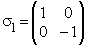Previous  Top  Next

Quaternions are similar to complex and hyper complex numbers, but their multiplication is not cummutative.
They have 3 non real parts, that are marked here with the letters j, k and l.
The real part will not have an own letter here.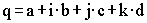The multiplication between the non real parts is defined in the following way: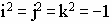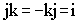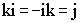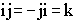Multiplication: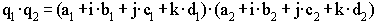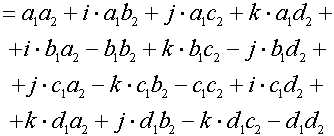<--compare hyper complex
Division: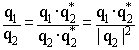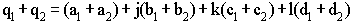Subtraction: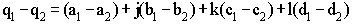conjunct:Abs: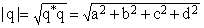InverseStandard quaternionDescription of rotation: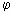corresponds to the rotation angle.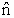corresponds to the rotation axis.
Power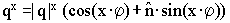A quaternion can also be written like a complexe 2x2-matrixor like a real 4x4-matrixQuaternions are mainly used in computer graphics to descibe rotations in the space.

Also they are used in quant mechanics to describe spins. Doing this, put instead of the marks (e,i,j,k) the Pauli spin matrices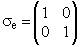;;;